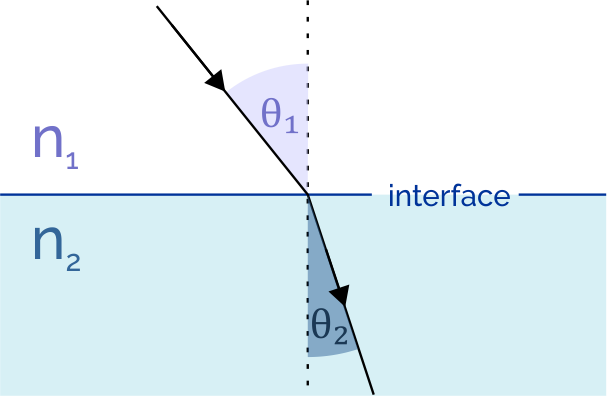# Angle of Refraction Calculator

Created by Michael Darcy
Reviewed by Dominik Czernia, PhD candidate
Last updated: Aug 31, 2022

Our angle of refraction calculator can help you understand why a spoon inside a partially filled glass appears oddly bent at the liquid's surface. We will show you how to use Snell's law to calculate the angle of refraction in water... and other substances because it's not the same for all materials. If you're curious to learn the reason for that and see the angle of refraction formula, read on!

## Angle of refraction definition

What is the angle of refraction? Imagine a ray of light crossing a surface - it will most likely come out deflected. The angle between the refracted wave and the surface normal is our quantity of interest.
A question arises: why is finding the angle of refraction helpful? Although it's rarely quoted on its own, you can substitute it into widely used Snell's law. Example applications include:

• Optical lenses, such as those used in eyeglasses. They effectively bend the light so that it hits your retina correctly.
• Medical imaging, for example, to account for ;
• Determining the refractive index of a media.

💡 Although we usually associate refraction angle with optics, Snell's law also works for other types of waves, for instance, sound.

## Angle of refraction formula

Now that we know the angle of refraction definition, it's time to learn the magic spell formula to calculate it.
The angle of refraction equation is derived from Snell's law, which describes the relationship between the refractive indices of both media and the angles of incidence and refraction. We can rearrange it as follows:

$\sin(\theta_2) = \frac{n_1\sin(\theta_1)}{n_2},$

where:

• $\theta_2$ - Angle of refraction;
• $\theta_1$ - Angle of incidence;
• $n_1$ - Refractive index of the medium from which the ray travels; and
• $n_2$ - Refractive index of the medium the light filters in.

Depending on the data, you might consider our angle conversion tool useful. It may be convenient if you need to know how to find the angle of refraction.

After computing the left-hand side, the last step in deriving the angle of refraction formula is taking the inverse sine:

$\theta_2 = \arcsin\left(\frac{n_1\sin(\theta_1)}{n_2} \right)$

## How do I calculate angle of refraction?

To find the angle of refraction:

1. Determine the refractive indices of both media the light passes through.
2. Establish the angle of incidence.
3. Divide the first substance's refractive index by the second medium's index of refraction.
4. Multiply the result by the sine of the incident angle.
5. Take the inverse sine of both sides to finish finding the angle of refraction.

If you'd like to learn more about Snell's law that we used to calculate the angle of refraction or any other quantities we used, check out our other calculators dealing with light-bending:

## FAQ

### How do I calculate the angle of refraction through glass?

Below points explain how to find the angle of refraction through glass:

1. Find the refractive index of air, n₁ = 1.000293.
2. Establish the glass refraction index, n₂ = 1.52.
3. Determine the angle of incidence, θ₁. We'll take it to be 45°.
4. Rearrange Snell's law to calculate the angle of refraction, θ₂: sin(θ₂) = n₁sin(θ₁)/n₂.
5. Substitute the known values to find sin(θ₂) = 0.465.
6. Solve for the angle of refraction by computing the inverse sine: θ₂ = arcsin(0.465) = 27.73°.

### Can the angle of refraction be 90°?

Yes, the angle of refraction can be 90°. It happens when the angle of incidence reaches its critical value - the highest magnitude for which the light isn't reflected.

Michael DarcyMedium 1
Vacuum
Refractive index 1 (n₁)
Medium 2
Air
Refractive index 2 (n₂)
Angle of incidence (θ₁)
deg
Angle of refraction (θ₂)
deg
People also viewed…

### BMR - Harris-Benedict equation

Harris-Benedict calculator uses one of the three most popular BMR formulas. Knowing your BMR (basal metabolic weight) may help you make important decisions about your diet and lifestyle.

### Generator power

The generator power calculator helps calculate the power requirement based on the current readings of various equipment and appliances or power supply voltage of the premises.

### Millionaire

This millionaire calculator will help you determine how long it will take for you to reach a 7-figure saving or any financial goal you have. You can use this calculator even if you are just starting to save or even if you already have savings.